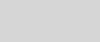# Using spatial geometry data in Azure Cosmos DBTim Sander

Many applications use spatial data to represent the physical locations and shapes of objects like cities, roads, and lakes. You can now store both the geography data type and the geometry data type in Azure Cosmos DB using the SQL (Core) API. The geography data type represents data in a round-earth coordinate system, and the geometry data type represents data in a Euclidean flat coordinate system.

Azure Cosmos DB supports indexing and querying of spatial data represented using the GeoJSON specification, and four spatial types:

• Point
• Polygon
• MultiPolygon
• LineString
##### Indexing geography data

This is a sample indexing policy that indexes Points, Polygons, MultiPolygons, and LineStrings with geography data indexing:

```{
"automatic":true,
"indexingMode":"Consistent",
"includedPaths": [
{
"path": "/*"
}
],
"spatialIndexes": [
{
"path": "/*",
"types": [
"Point",
"Polygon",
"MultiPolygon",
"LineString"
]
}
],
"excludedPaths":[]
}```
##### Indexing geometry data

Indexing geometry data is just like indexing geography data, but there are two additional steps that can be done in either the Azure Portal or Azure Cosmos DB .NET SDK.

1. Switch the geospatial configuration from geography (default) to geometry to let the Azure Cosmos DB query engine know what type of spatial data is being indexed. This is important because, though geography data and geometry data are both represented in GeoJSON, Azure Cosmos DB interprets and indexes the data differently, depending on the geospatial configuration.Setting geometry setting for spatial setting in Azure Cosmos DB

1. Add a bounding box to your indexing policy for each spatial path when switching to geometry spatial indexing. A bounding box isn’t necessary for geography spatial data because all geography data is based on its position on Earth and relevant GeoJSON types in valid latitude and longitude coordinates are always indexed. However, the two-dimensional Euclidean coordinate plane of geometry data does not have any limits, so you must specify a bounding box when you switch to geometry spatial indexing.
##### Using a bounding box

The bounding box consists of the following properties:

• xmin: the minimum indexed x coordinate
• ymin: the minimum indexed y coordinate
• xmax: the maximum indexed x coordinate
• ymax: the maximum indexed y coordinate

Create a bounding box that contains all (or most) of your data because only operations computed on the objects that are entirely inside the bounding box will be able to utilize the spatial index. Don’t make the bounding box significantly larger than necessary because doing so will negatively impact query performance. You can define the bounding box within your indexing policy like the example below in which all data that has an x coordinate between -10 and 10 and a y coordinate between -20 and 20 is indexed:

```           "boundingBox": {
"xmin": -10,
"ymin": -20,
"xmax": 10,
"ymax": 20
}```

When using spatial data, all possible types should be indexed. In Azure Cosmos DB, indexing additional paths can lead to a higher request unit (RU) charge for writes. However, only documents with valid GeoJSON paths have spatial indexes created. For example, if you only have Points in your dataset, then you can index both Points and Polygons for the same amount of RUs per write than if you’d just indexed Points.

##### Mixing spatial data with other types

Geography data and geometry data can’t be mixed within the same Azure Cosmos DB container, but spatial data can be mixed with other types of data. Spatial indexes can also be used alongside existing range and composite indexes. The same spatial system functions can be used with geography data and geometry data, including:

 ST_DISTANCE (spatial_expr, spatial_expr) Returns the distance between the two GeoJSON Point, Polygon, or LineString expressions. ST_WITHIN (spatial_expr, spatial_expr) Returns a Boolean expression indicating whether the first GeoJSON object (Point, Polygon, or LineString) is within the second GeoJSON object (Point, Polygon, or LineString). ST_INTERSECTS (spatial_expr, spatial_expr) Returns a Boolean expression indicating whether the two specified GeoJSON objects (Point, Polygon, or LineString) intersect. ST_ISVALID Returns a Boolean value indicating whether the specified GeoJSON Point, Polygon, or LineString expression is valid. ST_ISVALIDDETAILED Returns a JSON value containing a Boolean value if the specified GeoJSON Point, Polygon, or LineString expression is valid. If invalid, it returns the reason as a string value.

##### Get started

Learn more about using spatial data in Azure Cosmos DB: